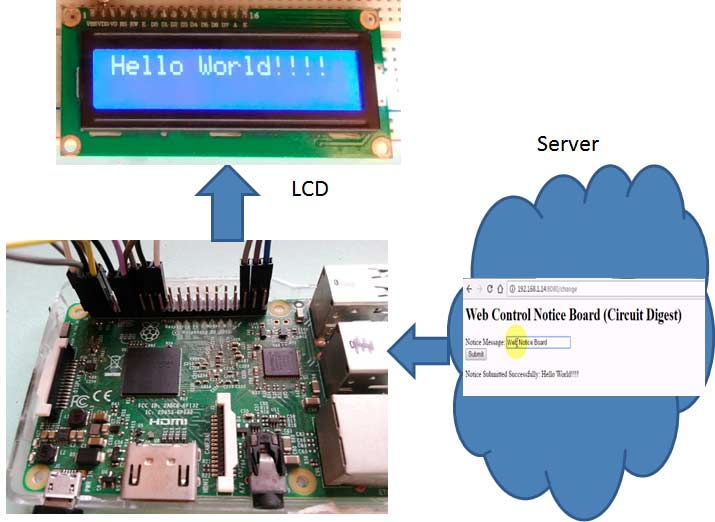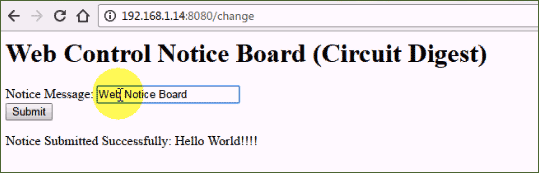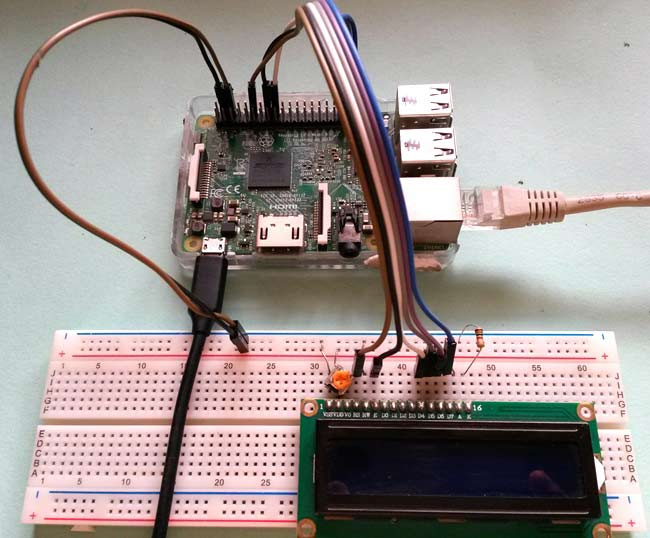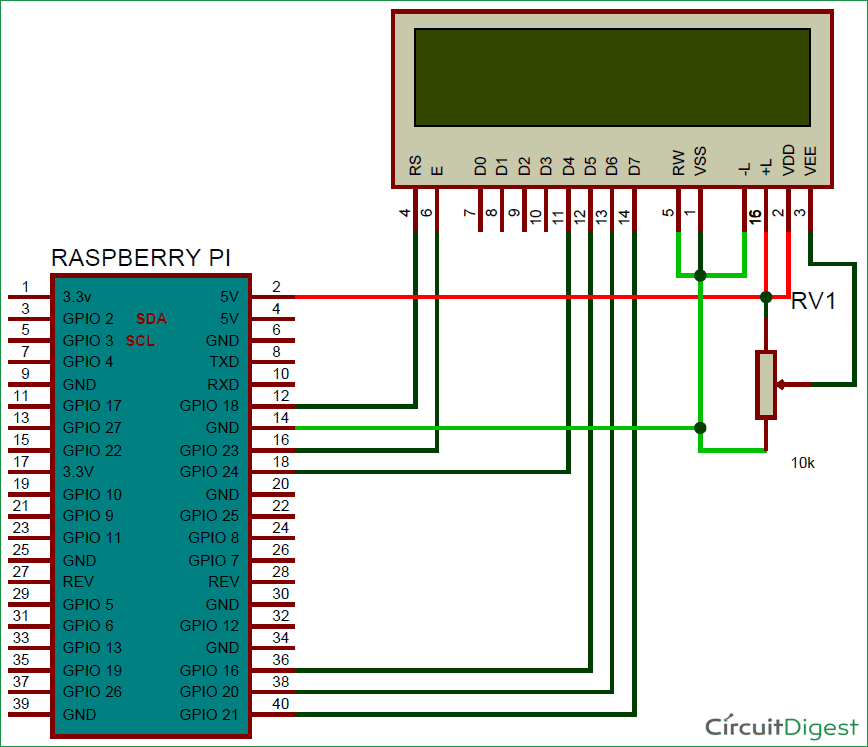We all are familiar with the Wireless Notice Board as we have already built Wireless Notice Board using GSM and Arduino. But today we are going one step ahead and instead of using GSM as wireless medium, this time we are using Internet to wirelessly send the message from Web Browser to the LCD which is connected to Raspberry Pi. As message is sent through the web browser, so it can be sent using Computer, smart phone or tablet, so it will add one more project in our IoT projects collection.

In this Web Controlled Notice Board, we have created a local web server for demonstration, this can be a global server over internet. At the Raspberry Pi, we have used 16x2 LCD to display message and Flask for receiving the message over network. Whenever Raspberry receives any wireless message from Web browser, it displays on the LCD. We will discuss about these things in detail in this article.

### Components Required:

• Raspberry Pi 3 (any model)
• Wi-Fi USB adapter (if not using Raspberry Pi 3)
• 16x2 LCD
• Power cable for Raspberry Pi
• Connecting wires
• 10K Pot

### Working Explanation and Creating the WebPage:

In this project, the main component is Raspberry Pi, which is the heart of this project and used to control the processes related to this project. Like: Driving LCD, receiving “Notice messages” from the server etc.Here, we have created a web server, which provides a way to send “Notice Message” to Raspberry Pi using Flask in a web browser. The Flask is a microframework for Python. This tool is Unicode based having built-in development server and debugger, integrated unit testing support, support for secure cookies and its easy to use, these things make it useful for the hobbyist.

We have created a webpage with a TextBox and a Submit button, where we can enter our “Notice Message” in TextBox and then submit it to the server by clicking on Submit button. This web application is developed by using HTML language. The code of this web page is given below and very easy to understand.

```<h1>Web Control Notice Board (Circuit Digest)</h1>
</div>

<div data-role="content">

<form method="post" action="change">
<label for="slider-1">Notice Message:</label>
<input type="text" name="lcd" id="lcd" />
<br />

<input type="submit" value="Submit" />
</form>

{% if value %}
<p>Notice Submitted Successfully: {{ value }}</p>
{% endif %}

</div>```User needs to copy-paste the above given HTML code in some text editor (notepad) and save the file with .HTML extension. Then put this HTML file in the same folder where you have put your Python Code file (given at the end) for this Web Controlled Notice Board. Now you can just run the Python code in Raspberry Pi, open the IP_address_of_your_Pi:8080 in web Browser (like 192.168.1.14:8080) and input the message and click submit, as soon as you submit the message, you will get the message on LCD connected to Raspberry Pi. Check the whole process in Demonstration Video at the end.The webpage is created using HTML language, which contain a form having a textbox and submit button, with Heading (h1 tag) Web Control Notice Board. The form has “change” is the action that will be performed in code using post method, when we click on Submit button. The slider is block with label “Notice Message”.

After it, we can add an optional line to show the text which we have sent to the Raspberry Pi via the server.

```{% if value %}
<p>Notice Submitted Successfully: {{ value }}</p>
{% endif %}```

It checks the value in the text box and if there is some value in the textbox then it prints the text on webpage itself, so that user can also see the submitted message. Here ‘value’ is “input text or notice message” that we will type in slider box or text box.

### Circuit Explanation:

Connections for this Wireless Message Board are very easy; we only need to connect LCD with the Raspberry Pi board by using some connectors over bread board. The user may use zero PCB for connections. RS, RW and EN pins of LCD are directly connected to pin 18, GND and 23. And data pins of LCD D4, D5, D6, D7 are directly connected to Raspberry Pi’s GPIO 24, 16, 20, 21. A 10K pot is used to control the brightness of LCD.Also remember, if you don’t have Raspberry Pi 3, you need to use the USB Wi-Fi adapter for lower version of Raspberry Pi as they don’t have inbuilt Wi-Fi like Raspberry Pi 3.

We are using Python language here for the Program. Before coding, user needs to configure Raspberry Pi. You can check our previous tutorials for Getting Started with Raspberry Pi and Installing & Configuring Raspbian Jessie OS in Pi.

Before program Raspberry Pi, user needs to install a flask support package into the Raspberry Pi by using given commands:

`\$ pip install Flask`

After it you can run the Python program in the python editor of Raspberry Pi but before this you need to replace the IP address in Program with IP address of your Raspberry Pi. You can check the IP address of your RPi board by using ifconfig command:

`Ifconfig`

The programming part of this project plays a very important role to perform all the operations. First of all, we include required libraries for Flask, initialize variables and define pins for LCD.

```from flask import Flask
import RPi.GPIO as gpio
import os, time

RS =18
EN =23
D4 =24
D5 =16
D6 =20
D7 =21
... ......
..... ......```

For LCD, def lcd_init() function is used to initialize LCD in four bit mode, def lcdcmd(ch) function is used for sending command to LCD, def lcddata(ch) function is used for sending data to LCD and def lcdstring(Str) function is used to send data string to LCD. You can check all these functions in Code given afterwards.

Below part of the Program is used to send the message from the web browser to Raspberry Pi using Flask. You can learn more about the programming using Flask here.

```@app.route("/")

def index():
return render_template('web.html')
@app.route("/change", methods=['POST'])

def change():
if request.method == 'POST':
# Getting the value from the webpage
data1 = request.form['lcd']
lcdcmd(0x01)
lcdprint(data1)
return render_template('web.html', value=data1)

if __name__ == "__main__":
app.debug = True
app.run('192.168.1.14', port=8080,debug=True)```

So this is how we can send the message from our computer or smartphone to the Raspberry Pi LCD and can make a IoT based Wireless Notice Board controlled over Web. Check the Full Python Code and Demonstration Video below.

Code

import RPi.GPIO as gpio
import os, time
RS =18
EN =23
D4 =24
D5 =16
D6 =20
D7 =21
HIGH=1
LOW=0
OUTPUT=1
INPUT=0
gpio.setwarnings(False)
gpio.setmode(gpio.BCM)
gpio.setup(RS, gpio.OUT)
gpio.setup(EN, gpio.OUT)
gpio.setup(D4, gpio.OUT)
gpio.setup(D5, gpio.OUT)
gpio.setup(D6, gpio.OUT)
gpio.setup(D7, gpio.OUT)
def begin():
lcdcmd(0x33)
lcdcmd(0x32)
lcdcmd(0x06)
lcdcmd(0x0C)
lcdcmd(0x28)
lcdcmd(0x01)
time.sleep(0.0005)

def lcdcmd(ch):
gpio.output(RS, 0)
gpio.output(D4, 0)
gpio.output(D5, 0)
gpio.output(D6, 0)
gpio.output(D7, 0)
if ch&0x10==0x10:
gpio.output(D4, 1)
if ch&0x20==0x20:
gpio.output(D5, 1)
if ch&0x40==0x40:
gpio.output(D6, 1)
if ch&0x80==0x80:
gpio.output(D7, 1)
gpio.output(EN, 1)
time.sleep(0.0005)
gpio.output(EN, 0)
# Low bits
gpio.output(D4, 0)
gpio.output(D5, 0)
gpio.output(D6, 0)
gpio.output(D7, 0)
if ch&0x01==0x01:
gpio.output(D4, 1)
if ch&0x02==0x02:
gpio.output(D5, 1)
if ch&0x04==0x04:
gpio.output(D6, 1)
if ch&0x08==0x08:
gpio.output(D7, 1)
gpio.output(EN, 1)
time.sleep(0.0005)
gpio.output(EN, 0)

def lcdwrite(ch):
gpio.output(RS, 1)
gpio.output(D4, 0)
gpio.output(D5, 0)
gpio.output(D6, 0)
gpio.output(D7, 0)
if ch&0x10==0x10:
gpio.output(D4, 1)
if ch&0x20==0x20:
gpio.output(D5, 1)
if ch&0x40==0x40:
gpio.output(D6, 1)
if ch&0x80==0x80:
gpio.output(D7, 1)
gpio.output(EN, 1)
time.sleep(0.0005)
gpio.output(EN, 0)
# Low bits
gpio.output(D4, 0)
gpio.output(D5, 0)
gpio.output(D6, 0)
gpio.output(D7, 0)
if ch&0x01==0x01:
gpio.output(D4, 1)
if ch&0x02==0x02:
gpio.output(D5, 1)
if ch&0x04==0x04:
gpio.output(D6, 1)
if ch&0x08==0x08:
gpio.output(D7, 1)
gpio.output(EN, 1)
time.sleep(0.0005)
gpio.output(EN, 0)

def lcdprint(Str):
l=0;
l=len(Str)
for i in range(l):
lcdwrite(ord(Str[i]))
begin()
lcdprint("Circuit Digest")
lcdcmd(0xc0)
lcdprint("Welcomes You")
time.sleep(5)
@app.route("/")
def index():
return render_template('web.html')
@app.route("/change", methods=['POST'])
def change():
if request.method == 'POST':
# Getting the value from the webpage
data1 = request.form['lcd']
lcdcmd(0x01)
lcdprint(data1)
return render_template('web.html', value=data1)
if __name__ == "__main__":
app.debug = True
app.run('192.168.1.14', port=8080,debug=True)

Video

Subscribe below to receive most popular news, articles and DIY projects from Circuit Digest

•Revathi
Dec 01, 2016

Notice board project is good

•Moses Hlauyo
May 25, 2017

I have done every step this project and it is showing funny characters on the lcd when type a simple message like "HelloWorld". How can i solve this issue

•mani teja
Jan 01, 2018

•tunhtutaye
Jun 01, 2017

Hello,nice to meet you.I want to know that can use native language in your Web Controlled IoT Notice Board using Raspberry Pi.

•Mani teja
Dec 28, 2017

sir i i want to have the code u shown in demonistration video,(which seems to be different one from that given in site).thank you in advance sir.please share me the code

•Mahesh h.r
Jan 13, 2018

nice

•Anurag
Feb 03, 2018

Sir, I just want to know that, Is there any proof or something that this project will work after its completion.

•Ashley
Mar 28, 2018

Sir, we have followed all the instructions given by you in the website but i was unable to open the web page when IP address is typed in the chrome.Can you help me to get web page opened?

•tanjina oriana
Jun 22, 2018

Sir, I have followed all your instruction but whenever I am trying to enter the webpage "jinja2.exceptions.TemplateNotFound: web.html" traceback is displayed and so I am unable to enter to the webpage. And while using 20*4 lcd instead of 16*2 lcd an OS error "OSError: [Errno 98] Address already in use" occured. Can you please help me.